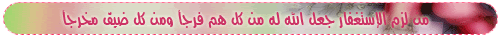###المحرر موضوع: Sorting Algorithms  (زيارة 1074 مرات)

0 الأعضاء و 1 ضيف يشاهدون هذا الموضوع.

أكتوبر 06, 2007, 08:25:03 مساءاً
زيارة 1074 مرات

#### mathematics##### Sorting Algorithms
« في: أكتوبر 06, 2007, 08:25:03 مساءاً »
Sorting Algorithms

Bubble sort
Heap sort
Insertion sort
Merge sort
Quick sort
Selection sort
Shell sort

One of the fundamental problems of computer science is ordering a list of items. There's a plethora of solutions to this problem, known as sorting algorithms. Some sorting algorithms are simple and intuitive, such as the bubble sort. Others, such as the quick sort are extremely complicated, but produce lightening-fast results.أكتوبر 06, 2007, 08:27:10 مساءاً
رد #1

#### mathematics##### Sorting Algorithms
« رد #1 في: أكتوبر 06, 2007, 08:27:10 مساءاً »
Bubble Sort

Algorithm Analysis
The bubble sort is the oldest and simplest sort in use. Unfortunately, it's also the slowest.
The bubble sort works by comparing each item in the list with the item next to it, and swapping them if required. The algorithm repeats this process until it makes a pass all the way through the list without swapping any items (in other words, all items are in the correct order). This causes larger values to "bubble" to the end of the list while smaller values "sink" towards the beginning of the list.
The bubble sort is generally considered to be the most inefficient sorting algorithm in common usage. Under best-case conditions (the list is already sorted), the bubble sort can approach a constant O(n) level of complexity. General-case is an abysmal O(n2).
While the insertion, selection, and shell sorts also have O(n2) complexities, they are significantly more efficient than the bubble sort.
.
Source Code
Below is the basic bubble sort algorithm.
CODE
void bubbleSort(int numbers[], int array_size)
{
int i, j, temp;

for (i = (array_size - 1); i >= 0; i--)
{
for (j = 1; j <= i; j++)
{
if (numbers[j-1] > numbers[j])
{
temp = numbers[j-1];
numbers[j-1] = numbers[j];
numbers[j] = temp;
}
}
}
}أكتوبر 06, 2007, 08:40:52 مساءاً
رد #2

#### mathematics##### Sorting Algorithms
« رد #2 في: أكتوبر 06, 2007, 08:40:52 مساءاً »
Heap Sort

Algorithm Analysis
The heap sort is the slowest of the O(n log n) sorting algorithms, but unlike the merge and quick sorts it doesn't require massive recursion or multiple arrays to work. This makes it the most attractive option for very large data sets of millions of items.
The heap sort works as it name suggests - it begins by building a heap out of the data set, and then removing the largest item and placing it at the end of the sorted array. After removing the largest item, it reconstructs the heap and removes the largest remaining item and places it in the next open position from the end of the sorted array. This is repeated until there are no items left in the heap and the sorted array is full. Elementary implementations require two arrays - one to hold the heap and the other to hold the sorted elements.
To do an in-place sort and save the space the second array would require, the algorithm below "cheats" by using the same array to store both the heap and the sorted array. Whenever an item is removed from the heap, it frees up a space at the end of the array that the removed item can be placed in.
Source Code
Below is the basic heap sort algorithm. The siftDown() function builds and reconstructs the heap.
CODE
void heapSort(int numbers[], int array_size)
{
int i, temp;

for (i = (array_size / 2)-1; i >= 0; i--)
siftDown(numbers, i, array_size);

for (i = array_size-1; i >= 1; i--)
{
temp = numbers;
numbers = numbers[i];
numbers[i] = temp;
siftDown(numbers, 0, i-1);
}
}

void siftDown(int numbers[], int root, int bottom)
{
int done, maxChild, temp;

done = 0;
while ((root*2 <= bottom) && (!done))
{
if (root*2 == bottom)
maxChild = root * 2;
else if (numbers[root * 2] > numbers[root * 2 + 1])
maxChild = root * 2;
else
maxChild = root * 2 + 1;

if (numbers[root] < numbers[maxChild])
{
temp = numbers[root];
numbers[root] = numbers[maxChild];
numbers[maxChild] = temp;
root = maxChild;
}
else
done = 1;
}
}أكتوبر 15, 2007, 01:14:05 صباحاً
رد #3

#### أميرة الأندلس##### Sorting Algorithms
« رد #3 في: أكتوبر 15, 2007, 01:14:05 صباحاً »
thanks alot, this will help me, i'll save it and read it, it's what we are studying these days..

i'm waiting for the next episode..: )
هوّن عليك فكل الأمر ينقطع ***** وخلّ عنك عنان الهم يندفع
فكل هم له من بعـده فرج ***** وكل أمر إذا ما ضاق يتسع
إن البلاء وإن طال الزمان به ***** فالموت يقطعه، أو سوف ينقطع

أكتوبر 27, 2007, 05:27:10 صباحاً
رد #4

#### أميرة الأندلس##### Sorting Algorithms
« رد #4 في: أكتوبر 27, 2007, 05:27:10 صباحاً »
where are you ?
هوّن عليك فكل الأمر ينقطع ***** وخلّ عنك عنان الهم يندفع
فكل هم له من بعـده فرج ***** وكل أمر إذا ما ضاق يتسع
إن البلاء وإن طال الزمان به ***** فالموت يقطعه، أو سوف ينقطع